## Line

Eucliddefined a line as a breadthless length,'' and a straight line as a line which lies evenly with the points on itself'' (Kline 1956, Dunham 1990). Lines are intrinsically 1-dimensional objects, but may be embedded in higher dimensional Spaces. An infinite line passing through pointsand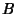is denoted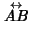. A Line Segment terminating at these points is denoted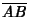. A line is sometimes called a Straight Line or, more archaically, a Right Line (Casey 1893), to emphasize that it has no curves anywhere along its length.

Consider first lines in a 2-D Plane. The line with x-Interceptand y-Intercept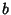is given by the intercept form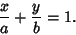(1)

The line through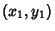with Slopeis given by the point-slope form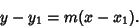(2)

The line with-interceptand slopeis given by the slope-intercept form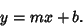(3)

The line throughandis given by the two point form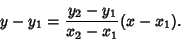(4)

Other forms are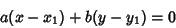(5)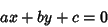(6)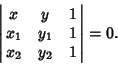(7)

A line in 2-D can also be represented as a Vector. The Vector along the line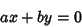(8)

is given by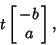(9)

where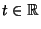. Similarly, Vectors of the form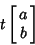(10)

are Perpendicular to the line. Three points lie on a line if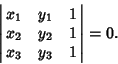(11)

The Angle between lines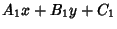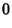(12)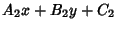(13)

is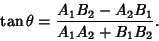(14)

The line joining points with Trilinear Coordinates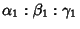and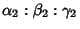is the set of point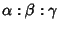satisfying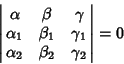(15)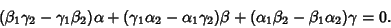(16)

Three lines Concur if their Trilinear Coordinates satisfy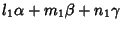(17)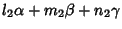(18)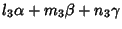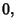(19)

in which case the point is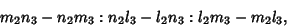(20)

or if the Coefficients of the lines(21)(22)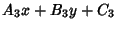(23)

satisfy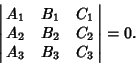(24)

Two lines Concur if their Trilinear Coordinates satisfy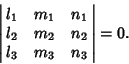(25)

The line through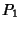is the direction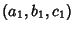and the line through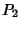in direction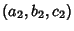intersect Iff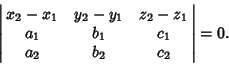(26)

The line through a point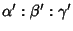Parallel to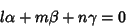(27)

is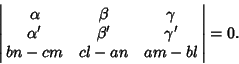(28)

The lines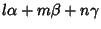(29)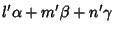(30)

are Parallel if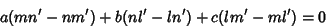(31)

for all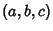, and Perpendicular if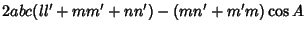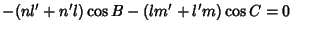(32)
for all(Sommerville 1924). The line through a pointPerpendicular to (32) is given by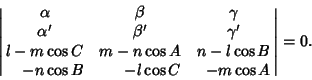(33)

In 3-D Space, the line passing through the point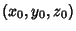and Parallel to the Nonzero Vector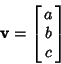(34)

has parametric equations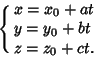(35)

See also Asymptote, Brocard Line, Collinear, Concur, Critical Line, Desargues' Theorem, Erdös-Anning Theorem, Line Segment, Ordinary Line, Pencil, Point, Point-Line Distance--2-D, Point-Line Distance--3-D, Plane, Range (Line Segment), Ray, Solomon's Seal Lines, Steiner Set, Steiner's Theorem, Sylvester's Line Problem

References

Casey, J. The Right Line.'' Ch. 2 in A Treatise on the Analytical Geometry of the Point, Line, Circle, and Conic Sections, Containing an Account of Its Most Recent Extensions, with Numerous Examples, 2nd ed., rev. enl. Dublin: Hodges, Figgis, & Co., pp. 30-95, 1893.

Dunham, W. Journey Through Genius: The Great Theorems of Mathematics. New York: Wiley, p. 32, 1990.

Kline, M. The Straight Line.'' Sci. Amer. 156, 105-114, Mar. 1956.

MacTutor History of Mathematics Archive. Straight Line.'' http://www-groups.dcs.st-and.ac.uk/~history/Curves/Straight.html.

Sommerville, D. M. Y. Analytical Conics. London: G. Bell, p. 186, 1924.

Spanier, J. and Oldham, K. B. The Linear Function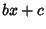and Its Reciprocal.'' Ch. 7 in An Atlas of Functions. Washington, DC: Hemisphere, pp. 53-62, 1987.